# MAFS.5.NF.1.2Archived StandardExport Print
Solve word problems involving addition and subtraction of fractions referring to the same whole, including cases of unlike denominators, e.g., by using visual fraction models or equations to represent the problem. Use benchmark fractions and number sense of fractions to estimate mentally and assess the reasonableness of answers. For example, recognize an incorrect result 2/5 + 1/2 = 3/7, by observing that 3/7 < 1/2.

### Remarks

Examples of Opportunities for In-Depth Focus

When students meet this standard, they bring together the threads of fraction equivalence (grades 3–5) and addition and subtraction (grades K–4) to fully extend addition and subtraction to fractions.
General Information
Subject Area: Mathematics
Domain-Subdomain: Number and Operations - Fractions
Cluster: Level 2: Basic Application of Skills & Concepts
Cluster: Use equivalent fractions as a strategy to add and subtract fractions. (Major Cluster) -

Clusters should not be sorted from Major to Supporting and then taught in that order. To do so would strip the coherence of the mathematical ideas and miss the opportunity to enhance the major work of the grade with the supporting clusters.

Date of Last Rating: 02/14
Status: State Board Approved - Archived
Assessed: Yes
Test Item Specifications

• Assessment Limits :
Fractions greater than 1 and mixed numbers may be included. Expressions may have up to three terms. Least common denominator is not necessary to calculate sums or differences of fractions. Items may not use the terms “simplify” or “lowest terms.” For given fractions in items, denominators are limited to 1-20. Items may require the use of equivalent fractions to find a missing term or part of a term.
• Calculator :

No

• Context :

Required

Sample Test Items (4)
• Test Item #: Sample Item 1
• Question:

John and Sue are baking cookies. The recipe lists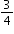cup of flour. They only have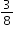cup of flour left.

How many more cups of flour do they need to bake the cookies?

• Difficulty: N/A
• Type: EE: Equation Editor

• Test Item #: Sample Item 2
• Question:

Javon, Sam, and Antoine are baking cookies. Javon has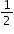cup of flour, Sam has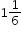cups of flour, and Antoine has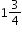cups of flour.

How many cups of flour do they have altogether?

• Difficulty: N/A
• Type: EE: Equation Editor

• Test Item #: Sample Item 3
• Question:

Richard and Gianni each bought a pizza. The pizzas are the same size.

• Richard cut his pizza into 12 slices.
• Gianni cut his pizza into 6 slices, and ate 2 slices.
• Together, Richard and Gianni ate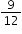How many slices of his pizza did Richard eat?

• Difficulty: N/A
• Type: MC: Multiple Choice

• Test Item #: Sample Item 4
• Question:

Jasmine hascup of flour in a mixing bowl. After adding more flour to the mixing bowl, Jasmine says that she now has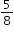cup of flour.

Which of the following explains why Jasmine's statement is incorrect?

• Difficulty: N/A
• Type: MC: Multiple Choice

## Related Courses

This benchmark is part of these courses.
5012070: Grade Five Mathematics (Specifically in versions: 2014 - 2015, 2015 - 2022, 2022 and beyond (current))
7712060: Access Mathematics Grade 5 (Specifically in versions: 2014 - 2015, 2015 - 2018, 2018 - 2022, 2022 and beyond (current))
5020120: STEM Lab Grade 5 (Specifically in versions: 2016 - 2022, 2022 and beyond (current))
5012065: Grade 4 Accelerated Mathematics (Specifically in versions: 2019 - 2022, 2022 and beyond (current))
5012015: Foundational Skills in Mathematics 3-5 (Specifically in versions: 2019 - 2022, 2022 and beyond (current))

## Related Access Points

Alternate version of this benchmark for students with significant cognitive disabilities.

## Related Resources

Vetted resources educators can use to teach the concepts and skills in this benchmark.

## Formative Assessments

Maris Has a Party:

Students are given a word problem involving fractions with unlike denominators and are asked to estimate the sum, explain their reasoning, and then determine the sum.

Type: Formative Assessment

Sarah’s Hike:

Students are asked to estimate the difference between two fractional lengths and then calculate the difference.

Type: Formative Assessment

Just Run:

Students are given a word problem involving subtraction of fractions with unlike denominators. Students are asked to determine if a given answer is reasonable, explain their reasoning, and calculate the answer.

Type: Formative Assessment

Baking Cakes:

Students are asked to estimate the sum of two mixed numbers and then calculate the sum.

Type: Formative Assessment

## Lesson Plans

Let's Have a Fraction Party!:

In this lesson, students will use addition and subtraction of fractions with unlike denominators to solve word problems involving situations that arise with the children who were invited to a party. They will use fraction strips as number models and connect the algorithm with these real-life word problems.

Type: Lesson Plan

Fractions make the real WORLD problems go round:

In this lesson students will use a graphic organizer to to solve addition and subtraction word problems. Students will create their own word problems in PowerPoint, by using pen and paper, or dry erase boards to help them to connect to and understand the structure of word problems.

Type: Lesson Plan

Aaron and Anya's Discovery: Adding Fractions with Unlike Denominators:

In this situational story, Aaron and Anya find several pieces of ribbon/cord of varying fractional lengths. They decide to choose 3 pieces and make a belt. All of the fractions have different denominators; students have to determine common denominators in order to add the fractional pieces. After students successfully add three fractional pieces, they make a belt and label it with their fractional pieces.

Type: Lesson Plan

Estimating Fractions Using Benchmark Fractions 0, 1/2, or 1:

In this lesson, students use models (fractions tiles or number lines) to round fractions using benchmark fractions of 0, 1/2, or 1.

Type: Lesson Plan

Discovering Common Denominators:

Students use pattern blocks to represent fractions with unlike denominators. Students discover that they need to convert the pattern blocks to the same size in order to add them. Therefore, they find and use common denominators for the addition of fractions.

Type: Lesson Plan

Looking for Patterns in a Sequence of Fractions:

Students generate and describe a numerical pattern using the multiplication and subtraction of fractions.

Type: Lesson Plan

## Original Student Tutorials

Making Art Part 2: Solving Addition and Subtraction Fraction Word Problems:

Learn to solve addition and subtraction word problems involving fractions with unlike denominators. As you complete this art-themed, interactive tutorial, you'll use visual models, write and solve equations, and check the reasonableness of results based on estimates.

This is part 2 of a two-part series. Click below to open part 1.

Type: Original Student Tutorial

Making Art Part 1: Estimating Adding and Subtracting Fractions Using Benchmarks:

Read word problems and use number lines with benchmarks to solve multi-step problems involving addition and subtraction of fractions with unlike denominators. In this tutorial, you will help Daisy and Angie paint pictures using fractions.

Type: Original Student Tutorial

Comparing two different pizzas:

The focus of this task is on understanding that fractions, in an explicit context, are fractions of a specific whole. In this this problem there are three different wholes: the medium pizza, the large pizza, and the two pizzas taken together. This task is best suited for instruction. Students can practice explaining their reasoning to each other in pairs or as part of a whole group discussion.

Jog-A-Thon:

The purpose of this task is to present students with a situation where it is natural to add fractions with unlike denominators; it can be used for either assessment or instructional purposes. Teachers should anticipate two types of solutions: one where students calculate the distance Alex ran to determine an answer, and one where students compare the two parts of his run to benchmark fractions.

The purpose of this task is to have students add fractions with unlike denominators and divide a unit fraction by a whole number. This accessible real-life context provides students with an opportunity to apply their understanding of addition as joining two separate quantities.

This task addresses common errors that students make when interpreting adding fractions word problems. It is very important for students to recognize that they only add fractions when the fractions refer to the same whole, and also when the fractions of the whole being added do not overlap. This set of questions is designed to enhance a student's understanding of when it is and is not appropriate to add fractions.

## Teaching Idea

Type: Teaching Idea

## MFAS Formative Assessments

Baking Cakes:

Students are asked to estimate the sum of two mixed numbers and then calculate the sum.

Just Run:

Students are given a word problem involving subtraction of fractions with unlike denominators. Students are asked to determine if a given answer is reasonable, explain their reasoning, and calculate the answer.

Maris Has a Party:

Students are given a word problem involving fractions with unlike denominators and are asked to estimate the sum, explain their reasoning, and then determine the sum.

Sarah’s Hike:

Students are asked to estimate the difference between two fractional lengths and then calculate the difference.

## Original Student Tutorials Mathematics - Grades K-5

Making Art Part 1: Estimating Adding and Subtracting Fractions Using Benchmarks:

Read word problems and use number lines with benchmarks to solve multi-step problems involving addition and subtraction of fractions with unlike denominators. In this tutorial, you will help Daisy and Angie paint pictures using fractions.

Making Art Part 2: Solving Addition and Subtraction Fraction Word Problems:

Learn to solve addition and subtraction word problems involving fractions with unlike denominators. As you complete this art-themed, interactive tutorial, you'll use visual models, write and solve equations, and check the reasonableness of results based on estimates.

This is part 2 of a two-part series. Click below to open part 1.

## Student Resources

Vetted resources students can use to learn the concepts and skills in this benchmark.

## Original Student Tutorials

Making Art Part 2: Solving Addition and Subtraction Fraction Word Problems:

Learn to solve addition and subtraction word problems involving fractions with unlike denominators. As you complete this art-themed, interactive tutorial, you'll use visual models, write and solve equations, and check the reasonableness of results based on estimates.

This is part 2 of a two-part series. Click below to open part 1.

Type: Original Student Tutorial

Making Art Part 1: Estimating Adding and Subtracting Fractions Using Benchmarks:

Read word problems and use number lines with benchmarks to solve multi-step problems involving addition and subtraction of fractions with unlike denominators. In this tutorial, you will help Daisy and Angie paint pictures using fractions.

Type: Original Student Tutorial

Comparing two different pizzas:

The focus of this task is on understanding that fractions, in an explicit context, are fractions of a specific whole. In this this problem there are three different wholes: the medium pizza, the large pizza, and the two pizzas taken together. This task is best suited for instruction. Students can practice explaining their reasoning to each other in pairs or as part of a whole group discussion.

Jog-A-Thon:

The purpose of this task is to present students with a situation where it is natural to add fractions with unlike denominators; it can be used for either assessment or instructional purposes. Teachers should anticipate two types of solutions: one where students calculate the distance Alex ran to determine an answer, and one where students compare the two parts of his run to benchmark fractions.

The purpose of this task is to have students add fractions with unlike denominators and divide a unit fraction by a whole number. This accessible real-life context provides students with an opportunity to apply their understanding of addition as joining two separate quantities.

This task addresses common errors that students make when interpreting adding fractions word problems. It is very important for students to recognize that they only add fractions when the fractions refer to the same whole, and also when the fractions of the whole being added do not overlap. This set of questions is designed to enhance a student's understanding of when it is and is not appropriate to add fractions.

## Parent Resources

Vetted resources caregivers can use to help students learn the concepts and skills in this benchmark.

Comparing two different pizzas:

The focus of this task is on understanding that fractions, in an explicit context, are fractions of a specific whole. In this this problem there are three different wholes: the medium pizza, the large pizza, and the two pizzas taken together. This task is best suited for instruction. Students can practice explaining their reasoning to each other in pairs or as part of a whole group discussion.

Jog-A-Thon:

The purpose of this task is to present students with a situation where it is natural to add fractions with unlike denominators; it can be used for either assessment or instructional purposes. Teachers should anticipate two types of solutions: one where students calculate the distance Alex ran to determine an answer, and one where students compare the two parts of his run to benchmark fractions.